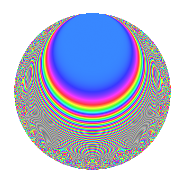# Properties

 Label 403.2.f.bLevel 403 Weight 2 Character orbit 403.f Analytic conductor 3.218 Analytic rank 0 Dimension 34 CM No

# Related objects

## Newspace parameters

 Level: $$N$$ = $$403 = 13 \cdot 31$$ Weight: $$k$$ = $$2$$ Character orbit: $$[\chi]$$ = 403.f (of order $$3$$ and degree $$2$$)

## Newform invariants

 Self dual: No Analytic conductor: $$3.21797120146$$ Analytic rank: $$0$$ Dimension: $$34$$ Relative dimension: $$17$$ over $$\Q(\zeta_{3})$$ Sato-Tate group: $\mathrm{SU}(2)[C_{3}]$

## $q$-expansion

The dimension is sufficiently large that we do not compute an algebraic $$q$$-expansion, but we have computed the trace expansion.

 $$\operatorname{Tr}(f)(q) =$$ $$34q + 4q^{2} - 20q^{4} - 14q^{5} + 6q^{6} + 6q^{7} - 12q^{8} - 17q^{9} + O(q^{10})$$ $$\operatorname{Tr}(f)(q) =$$ $$34q + 4q^{2} - 20q^{4} - 14q^{5} + 6q^{6} + 6q^{7} - 12q^{8} - 17q^{9} - 6q^{10} + 13q^{11} + 8q^{12} - 3q^{13} + 4q^{15} - 34q^{16} + 6q^{17} + 24q^{18} + 4q^{19} + 28q^{20} - 36q^{21} + 34q^{22} + 8q^{23} + 40q^{24} + 16q^{25} - 26q^{26} - 6q^{27} + 21q^{28} + 6q^{29} - 19q^{30} - 34q^{31} + 6q^{32} + 7q^{33} - 48q^{34} + 9q^{35} + 14q^{37} + 22q^{38} - 21q^{39} - 20q^{40} + 43q^{41} - 33q^{42} - 18q^{43} - 56q^{44} + 26q^{45} + 7q^{46} - 12q^{47} + 95q^{48} + q^{49} + 44q^{50} + 52q^{51} - 24q^{52} - 10q^{53} + 27q^{54} - 39q^{55} - 39q^{56} - 92q^{57} + 8q^{58} - q^{59} - 42q^{60} + 19q^{61} - 4q^{62} + 5q^{63} + 84q^{64} - 32q^{65} + 52q^{66} + 10q^{67} - 34q^{68} - 32q^{69} + 48q^{70} + 35q^{71} - 26q^{72} - 22q^{73} + 68q^{74} + 62q^{75} + 2q^{76} + 42q^{77} - 81q^{78} + 2q^{79} + 49q^{80} - 37q^{81} - 35q^{82} - 48q^{83} - 34q^{84} - 13q^{85} - 152q^{86} + 22q^{87} + 37q^{88} + 42q^{89} + 30q^{90} - 39q^{91} + 30q^{92} - 42q^{94} - 34q^{95} - 66q^{96} - 38q^{97} + 8q^{98} - 34q^{99} + O(q^{100})$$

## Embeddings

For each embedding $$\iota_m$$ of the coefficient field, the values $$\iota_m(a_n)$$ are shown below.

For more information on an embedded modular form you can click on its label.

Label $$a_{2}$$ $$a_{3}$$ $$a_{4}$$ $$a_{5}$$ $$a_{6}$$ $$a_{7}$$ $$a_{8}$$ $$a_{9}$$ $$a_{10}$$
94.1 −1.36162 2.35840i 1.42643 + 2.47066i −2.70804 + 4.69046i −1.48160 3.88453 6.72821i 1.05061 1.81970i 9.30282 −2.56943 + 4.45038i 2.01739 + 3.49422i
94.2 −1.21866 2.11078i 0.165727 + 0.287048i −1.97025 + 3.41258i −0.0487430 0.403929 0.699625i 0.449852 0.779167i 4.72962 1.44507 2.50293i 0.0594010 + 0.102886i
94.3 −1.04303 1.80658i −0.534702 0.926131i −1.17583 + 2.03660i −1.36190 −1.11542 + 1.93197i −2.24250 + 3.88412i 0.733593 0.928187 1.60767i 1.42050 + 2.46038i
94.4 −0.806301 1.39655i −1.60737 2.78404i −0.300243 + 0.520037i −1.40674 −2.59204 + 4.48955i 1.36764 2.36882i −2.25686 −3.66725 + 6.35186i 1.13426 + 1.96459i
94.5 −0.579157 1.00313i −0.862835 1.49447i 0.329155 0.570113i 1.50607 −0.999433 + 1.73107i 0.722094 1.25070i −3.07916 0.0110326 0.0191090i −0.872252 1.51078i
94.6 −0.399878 0.692609i 0.607925 + 1.05296i 0.680195 1.17813i 2.33311 0.486192 0.842109i −0.0878879 + 0.152226i −2.68749 0.760853 1.31784i −0.932958 1.61593i
94.7 −0.0914916 0.158468i 0.877667 + 1.52016i 0.983259 1.70305i −3.62872 0.160598 0.278164i 1.84724 3.19952i −0.725806 −0.0405970 + 0.0703161i 0.331997 + 0.575036i
94.8 −0.0361865 0.0626768i 1.64544 + 2.84999i 0.997381 1.72751i 2.88575 0.119086 0.206262i −0.540773 + 0.936646i −0.289113 −3.91498 + 6.78094i −0.104425 0.180869i
94.9 0.0205421 + 0.0355799i −1.04665 1.81285i 0.999156 1.73059i 1.98920 0.0430008 0.0744796i 1.34392 2.32775i 0.164267 −0.690959 + 1.19678i 0.0408622 + 0.0707754i
94.10 0.466912 + 0.808715i 1.35923 + 2.35426i 0.563986 0.976853i −3.82118 −1.26928 + 2.19846i −2.11839 + 3.66916i 2.92098 −2.19501 + 3.80187i −1.78416 3.09025i
94.11 0.578965 + 1.00280i −0.235957 0.408690i 0.329599 0.570882i −3.25594 0.273222 0.473235i 0.0133059 0.0230465i 3.07917 1.38865 2.40521i −1.88508 3.26505i
94.12 0.600575 + 1.04023i 0.0302705 + 0.0524300i 0.278620 0.482584i 2.34059 −0.0363594 + 0.0629763i −0.828861 + 1.43563i 3.07163 1.49817 2.59490i 1.40570 + 2.43474i
94.13 0.948581 + 1.64299i −1.29227 2.23827i −0.799611 + 1.38497i −2.49083 2.45164 4.24636i −1.48428 + 2.57085i 0.760339 −1.83991 + 3.18681i −2.36275 4.09241i
94.14 1.11298 + 1.92773i −0.337463 0.584504i −1.47744 + 2.55900i 1.70140 0.751178 1.30108i 2.45364 4.24983i −2.12551 1.27224 2.20358i 1.89362 + 3.27984i
94.15 1.16646 + 2.02036i 0.786870 + 1.36290i −1.72124 + 2.98128i 2.09117 −1.83570 + 3.17953i −0.532652 + 0.922581i −3.36520 0.261670 0.453226i 2.43926 + 4.22492i
94.16 1.23104 + 2.13222i −1.38318 2.39574i −2.03090 + 3.51763i −1.87256 3.40549 5.89848i 1.18933 2.05998i −5.07632 −2.32637 + 4.02939i −2.30518 3.99270i
94.17 1.41028 + 2.44268i 0.400852 + 0.694296i −2.97779 + 5.15768i −2.47906 −1.13063 + 1.95831i 0.397709 0.688852i −11.1570 1.17864 2.04146i −3.49618 6.05556i
373.1 −1.36162 + 2.35840i 1.42643 2.47066i −2.70804 4.69046i −1.48160 3.88453 + 6.72821i 1.05061 + 1.81970i 9.30282 −2.56943 4.45038i 2.01739 3.49422i
373.2 −1.21866 + 2.11078i 0.165727 0.287048i −1.97025 3.41258i −0.0487430 0.403929 + 0.699625i 0.449852 + 0.779167i 4.72962 1.44507 + 2.50293i 0.0594010 0.102886i
373.3 −1.04303 + 1.80658i −0.534702 + 0.926131i −1.17583 2.03660i −1.36190 −1.11542 1.93197i −2.24250 3.88412i 0.733593 0.928187 + 1.60767i 1.42050 2.46038i
See all 34 embeddings
 $$n$$: e.g. 2-40 or 990-1000 Embeddings: e.g. 1-3 or 373.17 Significant digits: Format: Complex embeddings Normalized embeddings Satake parameters Satake angles

## Inner twists

This newform does not have CM; other inner twists have not been computed.

## Hecke kernels

This newform can be constructed as the kernel of the linear operator $$T_{2}^{34} - \cdots$$ acting on $$S_{2}^{\mathrm{new}}(403, [\chi])$$.